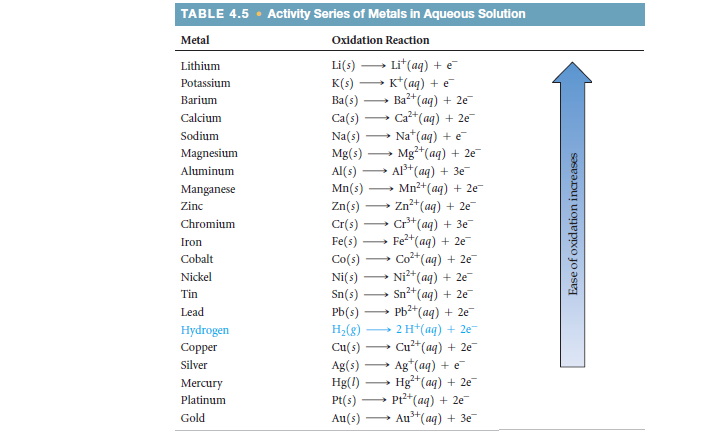# Problem: Using the activity series (Table 4.5), write balanced chemical equations for the following reactions. If no reaction occurs, simply write NR. (b) zinc metal is added to a solution of magnesium sulfate

###### FREE Expert Solution
83% (309 ratings)
###### FREE Expert Solution

We’re being asked to determine the outcome of the given reaction:

Zn(s) + MgSO4(aq)

Recall that the activity series gives us the relative reactivities of metals to oxidationmetals higher in the activity series are more reactive to oxidation and vice-versa.

83% (309 ratings)###### Problem Details

Using the activity series (Table 4.5), write balanced chemical equations for the following reactions. If no reaction occurs, simply write NR. (b) zinc metal is added to a solution of magnesium sulfate